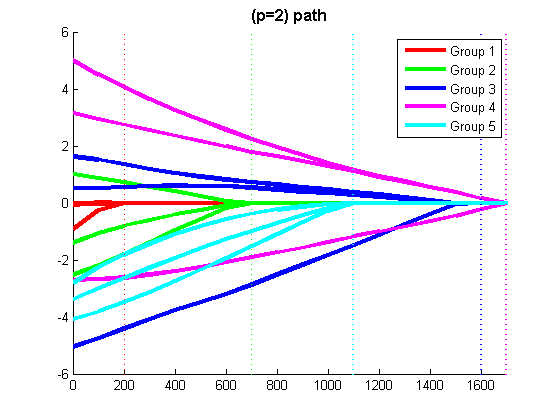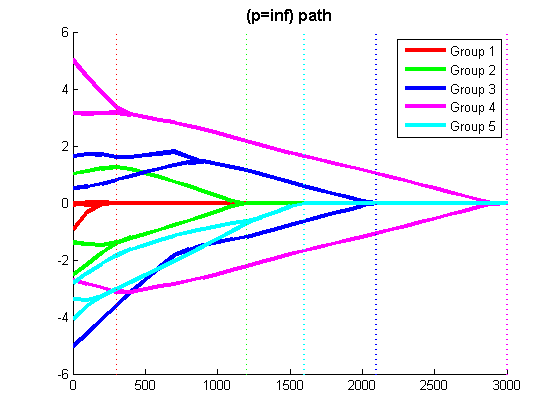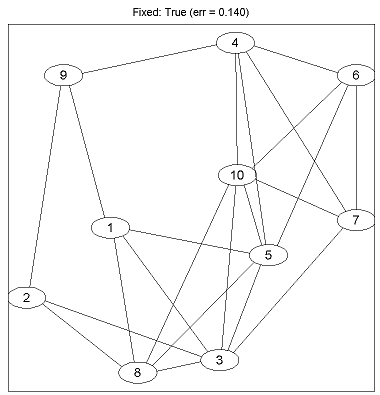# Matlab code for MAP estimation of pairwise Undirected Graphical Model structure with Group L1-Regularization

Written by Mark Schmidt and Kevin Murphy.
Last updated 7 September 2008.

# Summary

UGMlearn constains Matlab code for:
• Optimization of differentiable objective fucntions with Group L1-regularization (penalizing either the 2-norm or infinity-norm of the groups).
• MAP parameter and structure learning for probabilistic Undirected Graphical Models (UGMs) of discrete data with pairwise interactions (using Group-L1 regularization).

The algorithms are described in the following paper:

# Other related code

For code for MAP estimation of parameters and structure of directed acyclic models, see DAGlearn.
For code for solving (non-group) L1-regularization problems, see GeneralL1.

# How to use the code

```cd UGMlearn
mexAll
example_groupL1 % example of Group L1-Regularziation
example_UGMlearn(0) % example of Undirected Parameter/Structure learning methods
example_UGMlearn(1) % the same example, but showing the graphs (requires graphviz)
```

# Overview of the demo

The demo called by the above code illustrates the software's usage.

The script 'example_groupL1.m' generates a synthetic linear regression data set, and displays the Least Squares regularization path with L1-regularization, Group L1-regularization with the 2-norm, and Group L1-regularization with the infinity-norm (the script waits for the user to press a key between running the different methods).

The script 'example_UGMlearn.m' generates a synthetic conditional random field (CRF) data set, and runs the different parameter/structure learning methods from the paper on it. If called as 'example_UGMlearn(0)', it displays the test error produced by each method. If graphviz is installed, it can be called as 'example_UGMlearn(1)', and will display both the test error and final graph structures.

# Explanation of the GroupL1 Demo

These demos solve the problem:

min_w ||Xw - y||^2 + v sum_g ||w_g||_p

In the above, X is the 'n by p' design matrix, containing the p features for each of the n instances. y is the 'n by 1' regression target, and w is the 'p by 1' parameter vector that linearly transforms the rows of X to match the targets y. Each set g is subset of the parameters that we would like to penalize as a group. We obtain L1-regularization, Group L1-regularization with the 2-norms of the groups, and Group L1-regularization of the infinity-norms of the groups by setting the parameter p in the norm to 1, 2, or infinity (respectively). The strength of the regularization is controlled by the parameter v. The goal is to find the w that minimizes the squared error between Xw and y, subject to the regularization. Although this demo solves the (group) L1-regularization problem applied to this Least Squares objective, the optimization methods included in the code can be used to apply (group) L1-regularization to any continuously differentiable objective function.

Running the GroupL1 demo will produce plots similar to the three figures below (they may differ because of different random seeds). In these Figures, the x-axis indicates the scale of the regularizer, with larger values indicating a greater degree of regularization of the group norms. The y-axis shows values of the optimal parameters for a given regularization level. In this data set, the parameters and divided into 5 groups, indicated by colors. The vertical dotted lines are placed at x-axis locations where a variable becomes exactly 0, and the color of the vertical line indicates which group the variable that becomes exactly 0 belongs to. In the case of (p=1), notice that there are more than 1 vertical line for each group, and for a given regularization level each group might have some variables at zero and some variables non-zero. In contrast, when penalization of the (p=2) or (p=inf) group norm is used, there is only 1 vertical line for each group, since the variables in the same group are either all zero or all non-zero at the same time.

Below is the code used to generate the random data and specify the (sub) objective function (in this case Least Squares). Below each of the below plots we include the code needed to compute the parameters for a fixed regularization parameter.
```nInstances = 100; % Number of training examples
p = 15; % Number of variables
lambda = 500; % Strength of regularizer

% Make vector containing group memberships (note: Group 0 is reserved for non-regularized variables
groups = zeros(p,1);
groups(1:3:p) = [1:p/3]';
groups(2:3:p) = [1:p/3]';
groups(3:3:p) = [1:p/3]';
nGroups = length(unique(groups(groups>0)));

% Generate Data
X = randn(nInstances,p); % Generate Random Features
wTrue = randn(p,1).*groups; % Generate Random Weights, where higher groups have higher weight multiplier
y = X*wTrue + randn(nInstances,1); % Generate labels as linear combination plus random noise
funObj_sub = @(w)GaussianLoss(w,X,y); % We will be minimizing the Least Squares lost
``````% Code to optimize funObj_sub with L1-regularization
lambdaVect = lambda*ones(p,1); % Vector of regularization parameters (one for each variable)
funObj = @(w)nonNegGrad(w,lambdaVect,funObj_sub); % Surrogate objective function that divides variables into non-negative parts
wPosNeg = minConF_BC(funObj,zeros(2*p,1),zeros(2*p,1),inf(2*p,1)); % Solve non-negatively constrained optimization problem
w = wPosNeg(1:p)-wPosNeg(p+1:end) % Put non-negative parts together to get final anwser
``````% Code to optimize funObj_sub with Group L1-regularization (L1-regularization of group 2-norms)

% Make Auxiliary variable Objective and Projection Function
funObj = @(w)auxGroupLoss(w,groups,lambda,funObj_sub);
funProj = @(w)auxGroupL2Proj(w,groups);

% Solve
wAlpha = minConF_SPG(funObj,zeros(p+nGroups,1),funProj);
w = wAlpha(1:p) % Remove auxiliary variables
``````% Code to optimize funObj_sub with Group L1-regularization (L1-regularization of group infinity-norms)

% Make Auxiliary variable Objective and Projection Function
funObj = @(w)auxGroupLoss(w,groups,lambda,funObj_sub);
funProj = @(w)auxGroupLinfProj(w,groups);

% Solve
wAlpha = minConF_SPG(funObj,zeros(p+nGroups,1),funProj);
w = wAlpha(1:p) % Remove auxiliary variables
```

# Explanation of the UGMlearn demo

```nTrain = 500; % number of examples to use for training
nTest = 1000; % number of examples to use for testing
nFeatures = 10; % number of features for each node
nNodes = 10; % number of nodes
nStates = 2; % number of states that each node can take
```
The nTrain parameter specifies the number of examples to generate for training the CRF, and the nTest parameter specifies the number of examples used to evaluate its classification performance. The nFeatures parameter specifies the number of features associated with each node (in addition to the bias), it can be set to 0 in order to generate a Markov Random Field (MRF) instead of a CRF. nStates specifies the number of states that each node can take.
```edgeProb = .5; % probability of each edge being included in the graph
edgeType = 1; % set to 0 to make the edge features normally distributed
ising = 0; % set to 1 to use ising potentials
```
edgeProb specifies the probability that each edge is included in the underlying graph. Setting edgeType to 0 makes the edge parameters in the underlying graph normally distributed, and setting it to 1 makes the edge parameters uniformly distributed within an interval, where the interval size is normally distributed across the edges. Setting ising to 1 will use Ising-like potentials, while setting it to 0 will use full pairwise potentials.
```trainType = 'pseudo'; % set to 'pseudo' for pseudo-likelihood, 'loopy' for loopy belief propagation, 'exact' for 'exact inference
testType = 'exact'; % set to 'loopy' to test with loopy belief propagation, 'exact' for exact inference
structureSeed = 0; % change this to generate different structures
trainSeed = 0; % vary seed from 0:9 to get paper results
useMex = 1; % use mex files in UGM to speed things up
```
trainType can be set to 'pseudo' for training with pseudo-likelihood, 'loopy' for pseudo-moment matching with loopy belief propagation, or 'exact' for exact training (only suitable for up to ~16 nodes). testType can be set to 'exact' to use exact inference when testing (only suitable for up to ~16 nodes), and 'loopy' to test using loopy belief propagation for approximate inference. structureSeed is the seed passed to the random number generator when computing the graph structure, and trainSeed is the seed used when splitting the data into training and testing examples (the values 0:9 were used in the paper). Finally, setting useMex to 1 turns on the use of mex files to speed up parts of the computation in the UGM code, while setting it to 0 will exclusively use Matlab code.
```% Regularization Parameters
lambdaNode = 10;
lambdaEdge = 10;
```
These two parameters affect the strength of the regularizer. While the demo uses fixed values, in the paper we picked these values by two-fold cross-validation, testing the values 2.^[7:-1:-5], using warm-starting to speed up the optimization for this sequence of values.
```%% Generate data
nInstances = nTrain+nTest;
perm = randperm(nInstances);
trainNdx = perm(1:nTrain);
testNdx = perm(nTrain+1:end);
```
This code generates the synthetic CRF data, and splits it into training and testing examples.
```%% Fixed Structure Methods
edgePenaltyType = 'L2';

% Empty Structure
type = 'Fixed: Empty';
example_UGMlearnSub;
```
This code specifies that the edges are regularized with L2-regularization, and that the initial adjacency matrix is empty. example_UGMlearnSub.m is a script that trains and tests the CRF. The other 'Fixed Structure' and 'Generative Non-L1' methods are run by changing the adjInit matrix.
```%% Generative L1 Methods

% Regular L1
type = 'Generative L1: L1-L1';
edgePenaltyType = 'L1'; % Train with L1 regularization on the edges
Xold = X;
X = zeros(nInstances,0,nNodes); % Train while ignoring features
example_UGMlearnSub

X = Xold;
edgePenaltyType = 'L2';
subDisplay = display;
example_UGMlearnSub
```
For the 'Generative-L1' methods, we first train a full CRF that ignores the features and uses (group) L1-regularization in order to learn the graph structure. We then use this structure to train the final CRF that incorporates the features.
```%% Discriminative L1 Methods
type = 'Discriminative L1: L1-L1';
edgePenaltyType = 'L1'; % Train with L1 regularization on the edges
example_UGMlearnSub
```
For the 'Discriminative-L1' methods, we train a full CRF that uses the features and (group) L1-regularization in order to learn the parameters and graph structure at the same time.

Running the demo as 'example_UGMlearn(0)' will generate the following output:
```Generating Synthetic CRF Data...
Exact Sampling

Training Fixed: Empty...
Error Rate (Fixed: Empty): 0.266

Training Fixed: Chain...
Error Rate (Fixed: Chain): 0.250

Training Fixed: Full...
Error Rate (Fixed: Full): 0.184

Training Fixed: True...
Error Rate (Fixed: True): 0.140

Training Generative Non-L1: Tree...
Error Rate (Generative Non-L1: Tree): 0.220

Training Generative Non-L1: DAG...
Error Rate (Generative Non-L1: DAG): 0.224

Training Generative L1: L1-L1...
Error Rate (Generative L1: L1-L1): 0.204

Training Generative L1: L1-L2...
Error Rate (Generative L1: L1-L2): 0.202

Training Generative L1: L1-Linf...
Error Rate (Generative L1: L1-Linf): 0.192

Training Discriminative L1: L1-L1...
Error Rate (Discriminative L1: L1-L1): 0.180

Training Discriminative L1: L1-L2...
Error Rate (Discriminative L1: L1-L2): 0.150

Training Discriminative L1: L1-Linf...
Error Rate (Discriminative L1: L1-Linf): 0.178
```
Running the demo as 'example_UGMlearn(1)' will show the progress of the optimization algorithms and also pauses to show the final graph structure after each method is run. Below is the graph produced under the true generating structure: